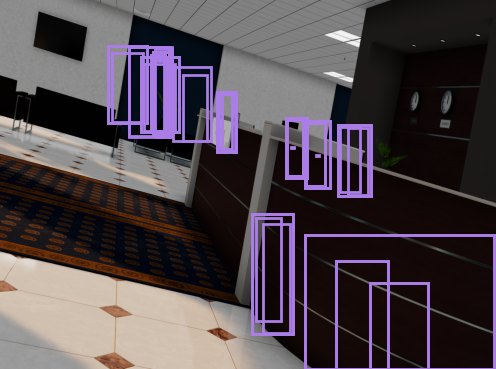# Visualization Bounding Box Image using script for offline generation replicator

Hi,

I’m using a custom offline generation to generate the synthetic data using replicator, however they only generate .npy and .json data for bounding boxes.
I want the bounding boxes data to be the .png images. Therefore, I tried to visualize it using codes with tutorial that I found here:
https://docs.omniverse.nvidia.com/prod_extensions/prod_extensions/ext_replicator/programmatic_visualization.html

However, I encounter some problem only on bounding boxes visualization. The generated rectangle was not in correct places.The boxes should be on TV, tables, etc with different color, but the bounding boxes detect different places with the same colors.
I appreciate if any of you could help me with this. Thank you :D

Hello @putri.riska! I’ve contacted the dev team for some assistance in answering your questions!

Hi Wendy,
Thank you for the prompt reply.
Could you help me out with visualizing 3D bounding boxes too?
I really appreciate it if you can.

1 Like

Hi @putri.riska! I just wanted to let you know that the dev team is investigating this. I’ve asked for an update from the team!

1 Like

Hi @putri.riska , did you assign different prims with different semantic labels? They will be different colors only if they have different semantic labels. Also, what kind of bbox data are you using? I just tested on my end and I think the visualization script works. Maybe there is some bug in the `bbox` data part.

Hi @jiehanw
I solved the issue. I used replicator generated bbox .npy data.
In the tutorial, it was written like this:

``````def colorize_bbox_2d(rgb_path, data, id_to_labels, file_path):

rgb_img = Image.open(rgb_path)
colors = [data_to_colour(bbox["semanticId"]) for bbox in data]
fig, ax = plt.subplots(figsize=(10, 10))
ax.imshow(rgb_img)
for bbox_2d, color, index in zip(data, colors, range(len(data))): #this one is the issue
labels = id_to_labels[str(index)]
rect = patches.Rectangle(
xy=(bbox_2d["x_min"], bbox_2d["y_min"]),
width=bbox_2d["x_max"] - bbox_2d["x_min"],
height=bbox_2d["y_max"] - bbox_2d["y_min"],
edgecolor=color,
linewidth=2,
label=labels,
fill=False,
)

plt.legend(loc="upper left")

plt.savefig(file_path)
``````

I changed the for loop part into:

``````    for bbox in data:
id = bbox["semanticId"]
color = data_to_colour(id)
labels = id_to_labels[str(id)]
rect = patches.Rectangle(
xy=(bbox["x_min"], bbox["y_min"]),
width=bbox["x_max"] - bbox["x_min"],
height=bbox["y_max"] - bbox["y_min"],
edgecolor=color,
linewidth=2,
label=labels,
fill=False,
)